aSc Substitutions - Specifying the substitutions

Using points to balance substitution/contract load

For each teacher you can specify his base weekly contract: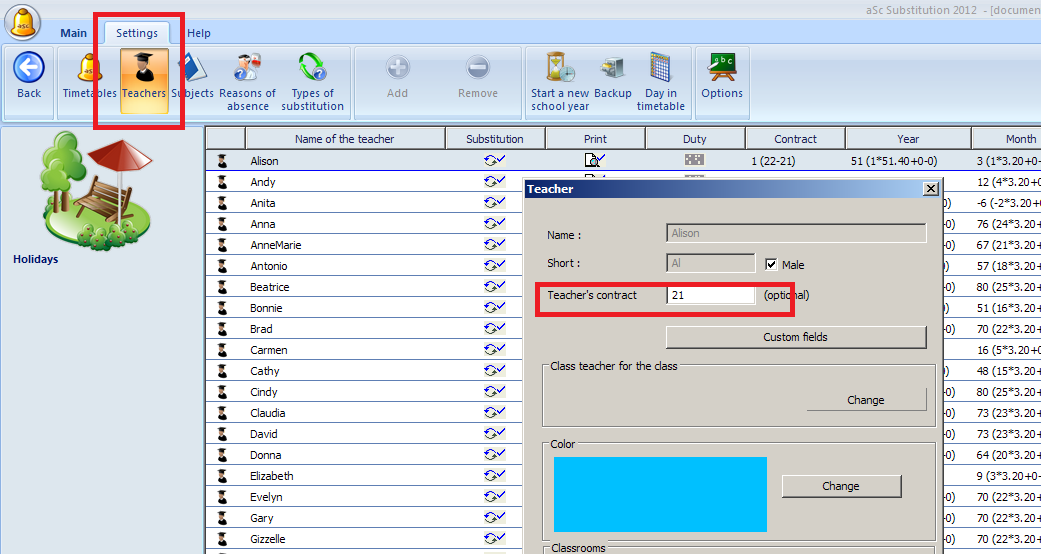The software then shows the difference between teacher's contract and his actual lessons: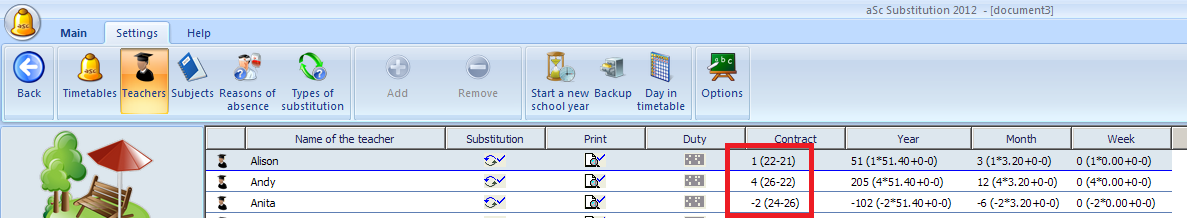If teacher is teaching more lessons per week than his contract is, he will receive 'plus' points each week.

Then for each reason of absence you can specify the point value. If this value is non zero, then each time a teacher is absent because of this reason, he/she will get negative points: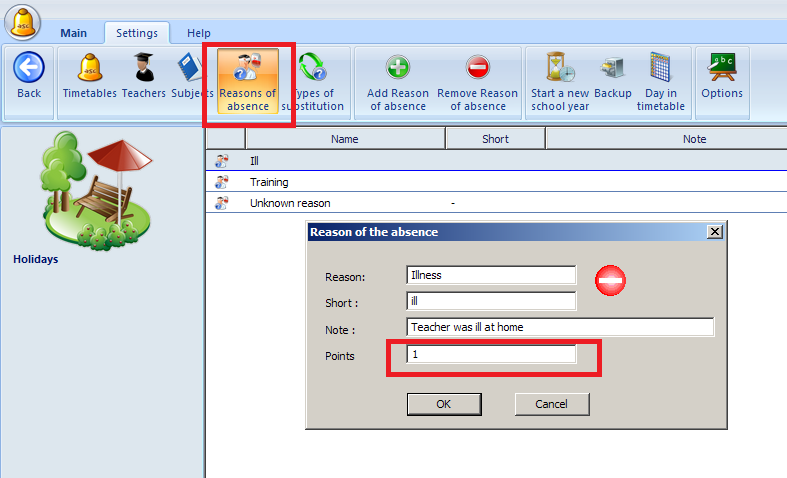Also for each type of substitution you can specify the points, and these then add positive points to the teacher's balance: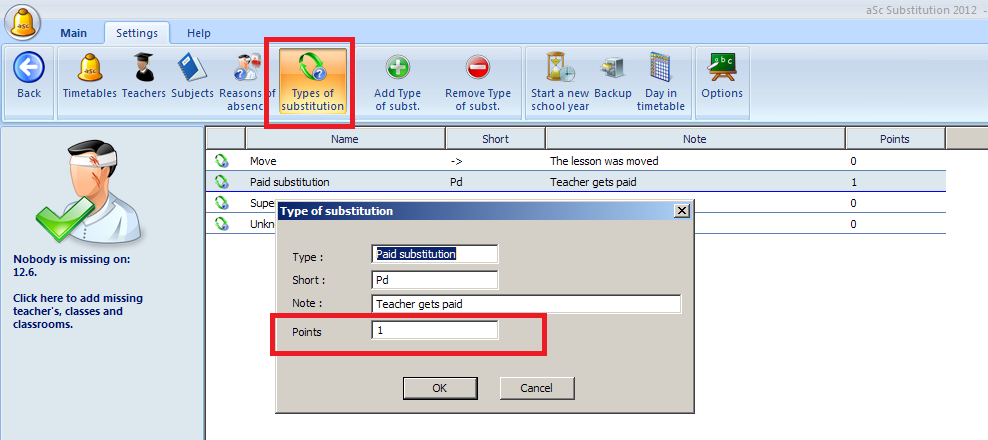The software will calculate for current week, current month and for the whole year the total points each teacher earned:and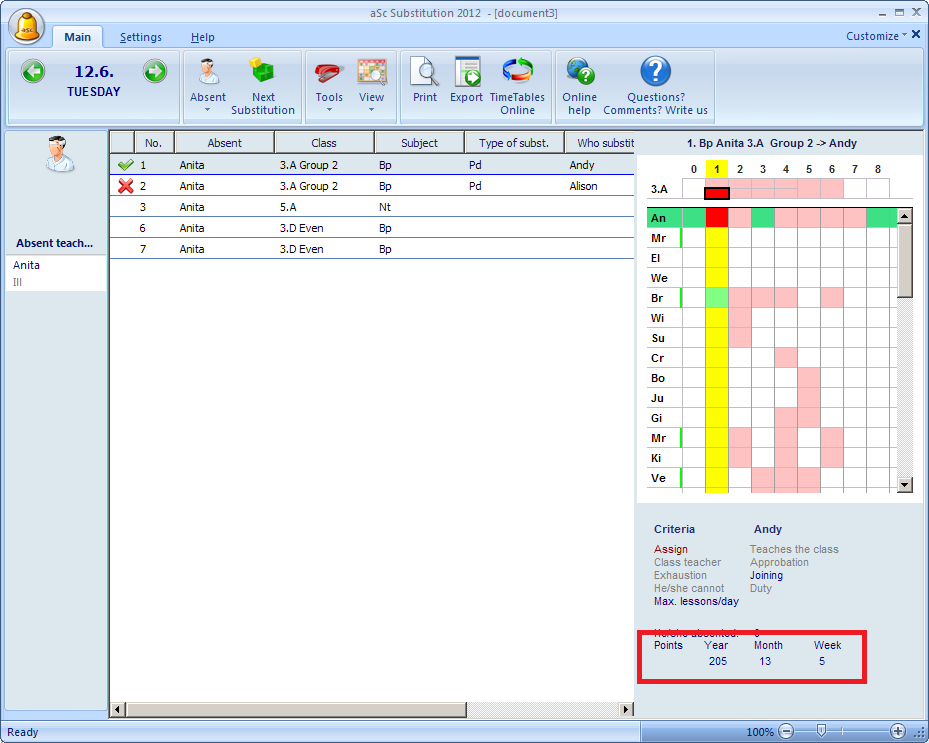The number is calculated as:

points for substitutions + points for weekly lessons over the contract - points for absences.

So in general the higher this number is the more this teacher has done for the school in the time frame. You can then use this number to balance each one to zero.

Notes:
- sometimes the numbers can be decimal, month can end in the middle of week, holidays are not counted etc.